• 十六进制转八进制，先转二进制然后再转八进制，通过StringBuffer和append();来完成衔接，详细的下载查看
• 蓝桥杯基础练习-进制转换（十六进制转八进制+十六进制转十进制+十进制转十六进制） 一、十六进制转八进制 试题 基础练习 十六进制转八进制 资源限制 时间限制：1.0s 内存限制：512.0MB 问题描述 　给定n个十六进制...
蓝桥杯基础练习-进制转换（十六进制转八进制+十六进制转十进制+十进制转十六进制）
一、十六进制转八进制
试题 基础练习 十六进制转八进制
资源限制
时间限制：1.0s   内存限制：512.0MB
问题描述
给定n个十六进制正整数，输出它们对应的八进制数。

输入格式
输入的第一行为一个正整数n （1<=n<=10）。
接下来n行，每行一个由0~9、大写字母A~F组成的字符串，表示要转换的十六进制正整数，每个十六进制数长度不超过100000。

输出格式
输出n行，每行为输入对应的八进制正整数。

【注意】
输入的十六进制数不会有前导0，比如012A。
输出的八进制数也不能有前导0。

样例输入
2
39
123ABC

样例输出
71
4435274

【提示】
先将十六进制数转换成某进制数，再由某进制数转换成八进制。

AC代码：
#include<bits/stdc++.h>
using namespace std;
string a={"0000","0001","0010","0011","0100","0101","0110","0111","1000","1001","1010","1011","1100","1101","1110","1111"};
string tran(char x)
{
string cnt="";
switch (x)
{
case '0':cnt+=a;break;
case '1':cnt+=a;break;
case '2':cnt+=a;break;
case '3':cnt+=a;break;
case '4':cnt+=a;break;
case '5':cnt+=a;break;
case '6':cnt+=a;break;
case '7':cnt+=a;break;
case '8':cnt+=a;break;
case '9':cnt+=a;break;
case 'A':cnt+=a;break;
case 'B':cnt+=a;break;
case 'C':cnt+=a;break;
case 'D':cnt+=a;break;
case 'E':cnt+=a;break;
case 'F':cnt+=a;break;
default:break;
}
return cnt;
}
int main(){
int n;
cin>>n;
string str;
for(int i=0;i<n;i++)
{
cin>>str[i];
string ans=""; //存放二进制字符串
int len=str[i].length();
for(int j=0;j<len;j++)
{
ans+=tran(str[i][j]);
}
int len2=ans.length();
if(len2%3==1)
{
ans="00"+ans;
}
else if(len2%3==2)
{
ans="0"+ans;
}
len2=ans.length();
string cnt=""; //存放八进制字符串
for(int j=0;j<len2;j+=3)
{
int k=(ans[j]-'0')*pow(2,2)+(ans[j+1]-'0')*pow(2,1)+(ans[j+2]-'0')*pow(2,0);
cnt+=(k+'0');
}
cnt.erase(0,cnt.find_first_not_of('0'));
cout<<cnt<<endl;
}
system("pause");
return 0;
}


二、十六进制转十进制
试题 基础练习 十六进制转十进制
资源限制
时间限制：1.0s   内存限制：512.0MB
问题描述
从键盘输入一个不超过8位的正的十六进制数字符串，将它转换为正的十进制数后输出。
注：十六进制数中的10~15分别用大写的英文字母A、B、C、D、E、F表示。
样例输入
FFFF
样例输出
65535

AC代码：
#include<bits/stdc++.h>
using namespace std;
int tran(char x)
{
int ans=0;
switch(x)
{
case '0':ans=0;break;
case '1':ans=1;break;
case '2':ans=2;break;
case '3':ans=3;break;
case '4':ans=4;break;
case '5':ans=5;break;
case '6':ans=6;break;
case '7':ans=7;break;
case '8':ans=8;break;
case '9':ans=9;break;
case 'A':ans=10;break;
case 'B':ans=11;break;
case 'C':ans=12;break;
case 'D':ans=13;break;
case 'E':ans=14;break;
case 'F':ans=15;break;
}
return ans;
}
int main(){
string str;
int a={0};
cin>>str;
int len=str.length();
long long int sum=0;
for(int i=len-1;i>=0;i--)
{
sum+=tran(str[i])*pow(16,len-i-1);
}
cout<<sum<<endl;
system("pause");
return 0;
}


三、十进制转十六进制
试题 基础练习 十进制转十六进制
资源限制
时间限制：1.0s   内存限制：512.0MB
问题描述
十六进制数是在程序设计时经常要使用到的一种整数的表示方式。它有0,1,2,3,4,5,6,7,8,9,A,B,C,D,E,F共16个符号，分别表示十进制数的0至15。十六进制的计数方法是满16进1，所以十进制数16在十六进制中是10，而十进制的17在十六进制中是11，以此类推，十进制的30在十六进制中是1E。
给出一个非负整数，将它表示成十六进制的形式。
输入格式
输入包含一个非负整数a，表示要转换的数。0<=a<=2147483647
输出格式
输出这个整数的16进制表示
样例输入
30
样例输出
1E

AC代码：
#include<bits/stdc++.h>
using namespace std;
char a={'0','1','2','3','4','5','6','7','8','9','A','B','C','D','E','F'};
int main(){
long long int n;
cin>>n;
long long int t=abs(n);
string str="";
if(t==0)
{
cout<<"0"<<endl;return 0;
}
while(t!=0)
{
int k=t%16;
str=a[k]+str;
t/=16;
}
cout<<str<<endl;
//system("pause");
return 0;
}




展开全文• 十六进制转八进制 问题描述 给定n个十六进制正整数，输出它们对应的八进制数。 输入格式 输入的第一行为一个正整数n （1<=n<=10）。 接下来n行，每行一个由09、大写字母AF组成的字符串，表示要转换的十六进制...
十六进制转八进制
问题描述
给定n个十六进制正整数，输出它们对应的八进制数。
输入格式
输入的第一行为一个正整数n （1<=n<=10）。
接下来n行，每行一个由09、大写字母AF组成的字符串，表示要转换的十六进制正整数，每个十六进制数长度不超过100000。
输出格式
输出n行，每行为输入对应的八进制正整数。
【注意】
输入的十六进制数不会有前导0，比如012A。
输出的八进制数也不能有前导0。
样例输入
2
39
123ABC
样例输出
71
4435274
【提示】
先将十六进制数转换成某进制数，再由某进制数转换成八进制。
代码如下：
import java.math.BigInteger;
import java.util.Scanner;

public class 十六进制转八进制 {
public static void main(String[] args) {
Scanner sc = new Scanner(System.in);
int n = sc.nextInt();
sc.nextLine();
for (int i = 0; i < n; i++) {
System.out.println(new BigInteger(sc.nextLine(), 16).toString(8));
}
}
}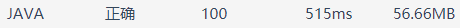十六进制转十进制
问题描述
从键盘输入一个不超过8位的正的十六进制数字符串，将它转换为正的十进制数后输出。
注：十六进制数中的10~15分别用大写的英文字母A、B、C、D、E、F表示。
样例输入
FFFF
样例输出
65535
代码如下：
Java
import java.util.Scanner;

public class 十六进制转十进制 {
public static void main(String[] args) {
Scanner sc = new Scanner(System.in);
String n = sc.nextLine();
System.out.println(Long.valueOf(n, 16));
}
}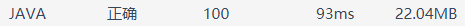C++
#include <iostream>
#include <cstdio>

using namespace std;

int main()
{
long long s;
scanf("%llx", &s);
printf("%lld", s);
return 0;
}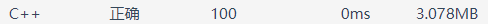十进制转十六进制
问题描述
十六进制数是在程序设计时经常要使用到的一种整数的表示方式。它有0,1,2,3,4,5,6,7,8,9,A,B,C,D,E,F共16个符号，分别表示十进制数的0至15。十六进制的计数方法是满16进1，所以十进制数16在十六进制中是10，而十进制的17在十六进制中是11，以此类推，十进制的30在十六进制中是1E。
给出一个非负整数，将它表示成十六进制的形式。
输入格式
输入包含一个非负整数a，表示要转换的数。0<=a<=2147483647
输出格式
输出这个整数的16进制表示
样例输入
30
样例输出
1E
代码如下：
Java
import java.util.Scanner;

public class 十进制转十六进制 {
public static void main(String[] args) {
Scanner sc = new Scanner(System.in);
int n = sc.nextInt();
System.out.println(Integer.toHexString(n).toUpperCase());//转为十六进制A---F大写
}
}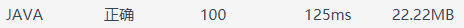C++
#include<iostream>
#include <cstdio>

using namespace std;

int main()
{
int n;
scanf("%d",&n);
printf("%X",n);
return 0;
}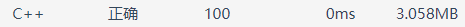小结：
①
十进制向二、八、十六进制的转换
Integer.toBinaryString(i)表示十进制转为二进制
Integer.toOctalString(i)表示十进制转为八进制
Integer.toHexString(i)表示十进制转为十六进制
②
进制转换问题可以直接用格式化输出。
十进制整数，%o表示以八进制进行输出
十进制整数，%X / %x表示以十六进制进行输出，大写X表示使用大写字母，小写x表示使用小写字母。
另外使用%#o,%#X,%#x,得到的输出包含前缀0,0X,0x。


展开全文• 基础练习十六进制转八进制时间限制：1.0s内存限制：512.0MB问题描述给定n个十六进制正整数，输出它们对应的八进制数。输入格式输入的第一行为一个正整数n(1<=n<=10)。接下来n行，每行一个由0~9、大写字母A~F...
基础练习 十六进制转八进制时间限制：1.0s   内存限制：512.0MB问题描述给定n个十六进制正整数，输出它们对应的八进制数。输入格式输入的第一行为一个正整数n (1<=n<=10)。接下来n行，每行一个由0~9、大写字母A~F组成的字符串，表示要转换的十六进制正整数，每个十六进制数长度不超过100000。输出格式输出n行，每行为输入对应的八进制正整数。注意输入的十六进制数不会有前导0，比如012A。输出的八进制数也不能有前导0。样例输入239123ABC样例输出714435274提示先将十六进制数转换成某进制数，再由某进制数转换成八进制。题解：先把十六进制转成二进制，每位十六进制正好转成4位二进制，然后再将二进制转成八进制，每三个二进制转成一个八进制。注意：在将16进制转成2进制时，要使用StringBuilder，否则会超时因为String每次修改字符串都是新建，然后删除旧的串会很影响效率，而StringBuilder是在字符串的末尾操作。代码：import java.util.Scanner;/**通过该类对象调用ChangToBit(char c)方法可返回2进制表示的字符串*/class Hexadecimal{String[] hex={"0000","0001","0010","0011","0100","0101","0110","0111","1000","1001","1010","1011","1100","1101","1110","1111"};public String ChangeToBit(char c){if(c>='0'&&c<='9'){return hex[c-'0'];}else return hex[c-'A'+10];}}/** 通过调用该类对象的changeToOex(String astring)方法可返回该3位2进制串的8进制数值*/class Oex{String[] oex={"000","001","010","011","100","101","110","111"};public int changeToOex(String astring){for(int i=0;i<8;i++){if(oex[i].equals(astring)==true){return i;}}return 0;}}public class Main {/*** @param args*/static Hexadecimal hex=new Hexadecimal();static Oex oex=new Oex();//将aString的位数在字符串开头补全为3的倍数，返回补全后的字符串public static String BuQuanSanWei(String aString){String ret="";if(aString.length()%3==0) return aString;if((aString.length()+1)%3==0){ret+="0";ret+=aString;return ret;}ret+="00";ret+=aString;return ret;}public static void main(String[] args) {Scanner scan=new Scanner(System.in);int N=scan.nextInt();while(N--!=0){String aString=scan.next();//必须用StringBuilder建立2进制字符串，否则由于需建立的字符太长会超时StringBuilder bitStringbuilder=new StringBuilder();for(int i=0;ibitStringbuilder.append(hex.ChangeToBit(aString.charAt(i)));}//补全位数为3的倍数String bitString=BuQuanSanWei(bitStringbuilder.toString());//标记是否有输出int hasOut=0;for(int i=0;iString nowCun="";nowCun+=bitString.charAt(i);nowCun+=bitString.charAt(i+1);nowCun+=bitString.charAt(i+2);int Num=oex.changeToOex(nowCun);if(i==0&&Num==0) continue;hasOut=1;System.out.print(Num);}if(hasOut==0) System.out.print("0");System.out.println();}}}
展开全文• 1.十六进制转八进制 见例题： 问题描述 　给定n个十六进制正整数，输出它们对应的八进制数。 输入格式 　输入的第一行为一个正整数n （1&lt;=n&lt;=10）。 　接下来n行，每行一个由09、大写字母AF组成的字符...
1.十六进制转八进制
见例题：
问题描述
给定n个十六进制正整数，输出它们对应的八进制数。
输入格式
输入的第一行为一个正整数n （1<=n<=10）。
接下来n行，每行一个由09、大写字母AF组成的字符串，表示要转换的十六进制正整数，每个十六进制数长度不超过100000。
输出格式
输出n行，每行为输入对应的八进制正整数。
【注意】
输入的十六进制数不会有前导0，比如012A。
输出的八进制数也不能有前导0。
样例输入
2
39
123ABC
样例输出
71
4435274
【提示】
先将十六进制数转换成某进制数，再由某进制数转换成八进制。
分析：多少了解点进制之间转换的朋友都知道 要用二进制作为中间值替换，即：先把十六进制转成二进制，每位十六进制正好转成4位二进制，然后再将二进制转成八进制，每三个二进制转成一个八进制。
那么用c++中的string类型或者java中的String类就更加的方便了，我本人对c++不是很熟，只自学了些基本的头文件和输入输出，大家可以看看这个链接：https://www.cnblogs.com/c1299401227/p/5370685.html
题解：
方法一：
适合数字比较小的情况下（显然就不适合这道题，所以我错了）：
16进制：%x，8进制：%o
#include<stdio.h>
#define ll long long
int main(){
int T,num;
scanf("%d",&T);
while(T--){
scanf("%x",&num);
printf("%o\n",num);
}
return 0;
}

方法二：
就是c++的string类型了：
转自：https://blog.csdn.net/qq_37962204/article/details/78628508
#include<string>
#include<cstdio>
#include<cmath>
#include<iostream>
using namespace std;
int main(){
int n;
string s1,s2;
cin >> n;
while(n--){
cin >> s1;
s2 = "";
for(int i = 0;i<s1.length();i++){
switch(s1[i]){
case '0':s2 += "0000";break;
case '1':s2 += "0001";break;
case '2':s2 += "0010";break;
case '3':s2 += "0011";break;
case '4':s2 += "0100";break;
case '5':s2 += "0101";break;
case '6':s2 += "0110";break;
case '7':s2 += "0111";break;
case '8':s2 += "1000";break;
case '9':s2 += "1001";break;
case 'A':s2 += "1010";break;
case 'B':s2 += "1011";break;
case 'C':s2 += "1100";break;
case 'D':s2 += "1101";break;
case 'E':s2 += "1110";break;
case 'F':s2 += "1111";break;
default:break;
}
}
//补成3的位数
if(s2.length()%3 == 1)
s2 = "00" + s2;
if(s2.length()%3 == 2)
s2 = "0" +s2;
int sum = 0;
int flag = 0;   //flag的作用就是防止最后输出前导零
for(int j = 0;j<s2.length()-2;j +=3){ //-2
//sum += 4*(s2[j+2]-'0') + 2*(s2[j+1]-'0') + (s2[j]-'0');
sum = 4*(s2[j]-'0') + 2*(s2[j+1]-'0') + (s2[j+2]-'0');
if(sum)
flag = 1;
if(flag)
cout << sum;
}
cout << endl;
}
return 0;
}


2.十六进制转十进制
法一：
#include<stdio.h>
typedef long long ll ;
int main(){
int num;
scanf("%x",&num);
printf("%lld\n",num);
return 0;
}

法二：转自：https://blog.csdn.net/zhangjianjun521/article/details/79081435
建议进入链接看完整
#include <stdio.h>
#include <string.h>

/* 十六进制数转换为十进制数 */
long hexToDec(char *source);

/* 返回ch字符在sign数组中的序号 */
int getIndexOfSigns(char ch);

int main()
{
char *hex = "75BCD15";

printf("16进制数：\t%s\n", hex);
printf("10进制数：\t%ld\n", hexToDec(hex));

return 0;
}

/* 十六进制数转换为十进制数 */
long hexToDec(char *source)
{
long sum = 0;
long t = 1;
int i, len;

len = strlen(source);
for(i=len-1; i>=0; i--)
{
sum += t * getIndexOfSigns(*(source + i));
t *= 16;
}

return sum;
}

/* 返回ch字符在sign数组中的序号 */
int getIndexOfSigns(char ch)
{
if(ch >= '0' && ch <= '9')
{
return ch - '0';
}
if(ch >= 'A' && ch <='F')
{
return ch - 'A' + 10;
}
if(ch >= 'a' && ch <= 'f')
{
return ch - 'a' + 10;
}
return -1;
}




展开全文• 十六进制转八进制：问题描述　给定n个十六进制正整数，输出它们对应的八进制数。输入格式　输入的第一行为一个正整数n （1&lt;=n&lt;=10）。　接下来n行，每行一个由0~9、大写字母A~F组成的字符串，表示要...
• 十六进制转八进制 解题思路 数列排序 解题思路 这几道题涉及到java进制转换的函数，一定要记住鸭~   十进制转十六进制 解题思路 import java.util.Scanner; public class Main { public static void ...java
• 　给定n个十六进制正整数，输出它们对应的八进制数。 输入格式 　输入的第一行为一个正整数n （1<=n<=10）。 　接下来n行，每行一个由09、大写字母AF组成的字符串，表示要转换的十六进制正整数，每个十六进制......